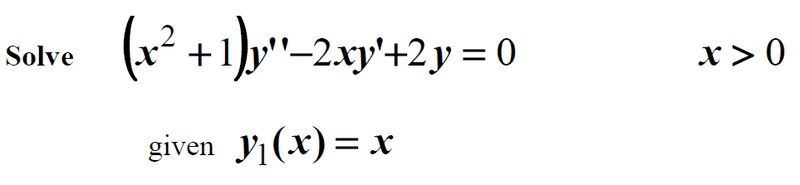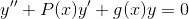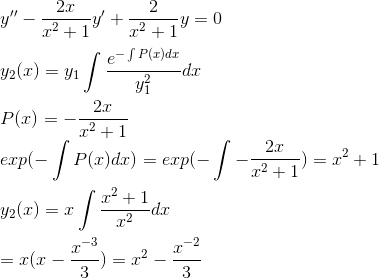# Solve the second-order differential equation

## Homework Statement## Homework Equations## The Attempt at a Solution#### Attachments

Buzz Bloom
Gold Member
Hi Fatima:

I suggest you take your solution
y(x) = x2 - (1/3)x-1
and calculate y'(x) and y"(x).
Then substitute these three functions of x in to corresponding terms of the original equation. After the substitution, the resulting equation should be reducible to
0 = 0​

Regards,
Buzz

the resulting equation should be reducible to
0 = 0
I didn't get this equation , can you tell me where is my mistake please ?

Mark44
Mentor

Mark44
Mentor
I didn't get this equation , can you tell me where is my mistake please ?
Your answer for ##y_2(x)## in the next-to-last line in post #1 is incorrect. Check the integration that you did.

Your answer for ##y_2(x)## in the next-to-last line in post #1 is incorrect. Check the integration that you did.
##y_2(x) = x(x-\frac{1}{x})##
##y_2(x) = x^2-1##
##y(x) = C_1 y_1(x) + C_2y_2(x)##
##y(x) = C1 x + C_2 (x^2-1)##

Mark44
Mentor
##y_2(x) = x(x-\frac{1}{x})##
##y_2(x) = x^2-1##
##y(x) = C_1 y_1(x) + C_2y_2(x)##
##y(x) = C1 x + C_2 (x^2-1)##
OK, now can you check that your solution is correct?

OK, now can you check that your solution is correct?
Got it , thank you.